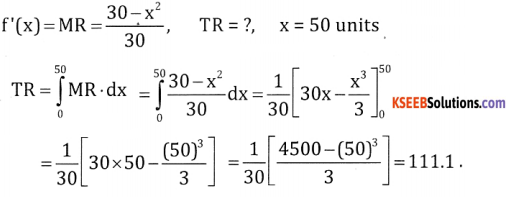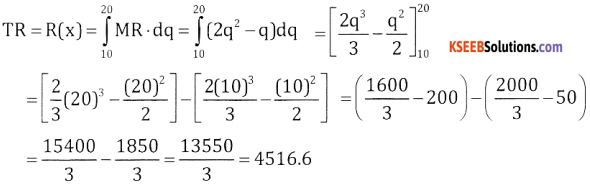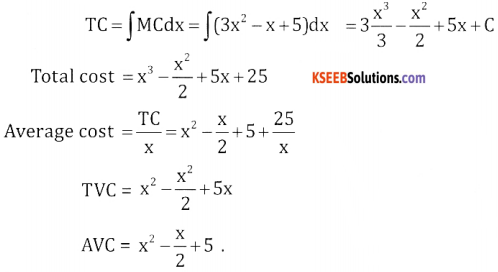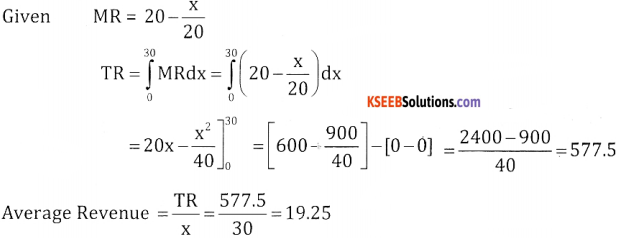# 2nd PUC Basic Maths Question Bank Chapter 21 Definite Integral and its Applications to Areas Ex 21.3

Students can Download Basic Maths Exercise 21.3 Questions and Answers, Notes Pdf, 2nd PUC Basic Maths Question Bank with Answers helps you to revise the complete Karnataka State Board Syllabus and score more marks in your examinations.

## Karnataka 2nd PUC Basic Maths Question Bank Chapter 21 Definite Integral and its Applications to Areas Ex 21.3

Part-A

Application of Integration to Economics

2nd PUC Basic Maths Definite Integral and its Applications to Areas Ex 21.3 Two Marks Questions and Answers

Question 1.
If the marginal cost of a firm is f'(x) = 10 + 6x – 6x2 where x is the output find the total cost assuming that the fixed cost is ₹ 125.
Given MC = f'(x) = 10 + 6x – 6x2, C = 125,TC = ?
TC = C(x) = ∫MCdx = ∫(10 +6x – 6x2)dx = 10x + $$\frac{6 x^{2}}{2}-\frac{6 x^{3}}{3}$$ + C
Total cost = 10x + 3x2 – 2x3 + 125.

Question 2.
If the marginal revenue is given by f'(x) = $$\frac{30-x^{2}}{30}$$. Find the revenue obtained from an output of 50 units.Question 3.
Find the total revenue in rupees by raising the output from 10 units to 20 units when the marginal revenue function is 2q2 – q where q is the output.
Given MR = 2q2 – q, TR = ?Part-B

2nd PUC Basic Maths Definite Integral and its Applications to Areas Ex 21.3 Three or five marks Questions and Answers

Question 1.
If the marginal cost function is 3×2 – x + 5 where x is the output, then find the total cost, average cost, total variable cost and average variable cost given that the fixed cost is ₹ 25.If the marginal revenue is f'(x) = $$20-\frac{x}{20}$$ Find the total revenue and average revenue obtained from an output of 30 units.